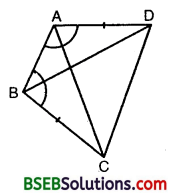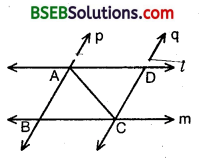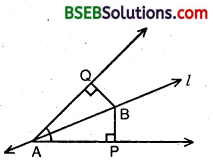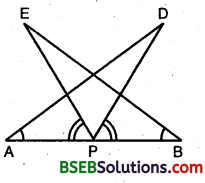# HSSlive: Plus One & Plus Two Notes & Solutions for Kerala State Board

## BSEB Class 9 Maths Chapter 7 Triangles Ex 7.1 Textbook Solutions PDF: Download Bihar Board STD 9th Maths Chapter 7 Triangles Ex 7.1 Book AnswersBSEB Class 9 Maths Chapter 7 Triangles Ex 7.1 Textbook Solutions PDF: Download Bihar Board STD 9th Maths Chapter 7 Triangles Ex 7.1 Book Answers

BSEB Class 9th Maths Chapter 7 Triangles Ex 7.1 Textbooks Solutions and answers for students are now available in pdf format. Bihar Board Class 9th Maths Chapter 7 Triangles Ex 7.1 Book answers and solutions are one of the most important study materials for any student. The Bihar Board Class 9th Maths Chapter 7 Triangles Ex 7.1 books are published by the Bihar Board Publishers. These Bihar Board Class 9th Maths Chapter 7 Triangles Ex 7.1 textbooks are prepared by a group of expert faculty members. Students can download these BSEB STD 9th Maths Chapter 7 Triangles Ex 7.1 book solutions pdf online from this page.

## Bihar Board Class 9th Maths Chapter 7 Triangles Ex 7.1 Books Solutions

 Board BSEB Materials Textbook Solutions/Guide Format DOC/PDF Class 9th Subject Maths Chapter 7 Triangles Ex 7.1 Chapters All Provider Hsslive

## How to download Bihar Board Class 9th Maths Chapter 7 Triangles Ex 7.1 Textbook Solutions Answers PDF Online?

2. Click on the Bihar Board Class 9th Maths Chapter 7 Triangles Ex 7.1 Answers.
3. Look for your Bihar Board STD 9th Maths Chapter 7 Triangles Ex 7.1 Textbooks PDF.
4. Now download or read the Bihar Board Class 9th Maths Chapter 7 Triangles Ex 7.1 Textbook Solutions for PDF Free.

Find below the list of all BSEB Class 9th Maths Chapter 7 Triangles Ex 7.1 Textbook Solutions for PDF’s for you to download and prepare for the upcoming exams:

## BSEB Bihar Board Class 9th Maths Solutions Chapter 7 Triangles Ex 7.1

Question 1.
In quadrilateral ACBD, AC = AD and AB bisects ∠A (see figure). Show that ∆ ABC ≅ ∆ ABD. What can you say about BC and BD?Solution:
Now, in As ABC and ABD, we have
∠CAB = ∠BAD (∵ AB bisects ∠A]
and, AB = AB [Common]
∴ By SAS congruence criterion, we have
∆ ABC ≅ ∆ ABD
⇒ BC = BD
[∵ Corresponding parts of congruent triangles are equal]

Question 2.
ABCD is a quadrilateral in which AD = BC and ∠DAB = ∠CBA (see figure). Prove that
(i) ∆ ABD ≅ ∆BAC
(ii) BD = AC
(iii) ∠ABD = ∠BAC.Solution:
In ∆s ABD and BAC, we have
∠DAB = ∠CBA lGiven]
AB = AB [Common]
By SAS criterion of congruence, we have A ABD = A BAC, which proves (i)
⇒ BD = AC
and, ∠ABD = ∠BAC, which proves (ii) and (iii)
[∵ Corresponding parts of congruent triangles are equal]

Question 3.
AD and BC are equal „ perpendiculars to a line segment AB (see figure). Show that CD bisects AB.Solution:
Since AB and CD intersect at O. Therefore,
∠AOD =∠BOC … (1) [Vertically opp. angles]
In ∆s AOD and BOC, we have
∠AOD = ∠BOC [From(1)]
∠D AO = ∠OBG [Each = 90°]
∴ By AAS congruence criterion, we have ∆ AOD ≅ ∆ BOC
OA = OB
[∵ Corresponding parts of congruent triangles are equal] i.e., O is the mid-point AB.
Hence, CD bisects AB.

Question 4.
1 and m are two parallel lines intersected by another pair of parallel lines p and q (see figure). Show that ∆ ABC ≅ ∆ CDA.Solution:
Since l and m are two parallel lines intersected by another pair of parallel lines p and q. Therefore, AD || BC and AB || CD ⇒ ABCD is a parallelogram.
i.e., AB = CD
Now, in ∆s ABC and CDA, we have
AB = CD [Prove above]
and AC = AC [Common]
∴ By SSS criterion of congruence ∆ ABC ≅ ∆ CDA.

Question 5.
Line l is the bisector of an angle A and B is any point on l. BP and BQ are perpendiculars from B to the arms of ∠A (see figure). Show that :
(i) ∆ APB ≅ ∆ AQB
(ii) BP = BQ or B is equidistant from the arms of ∠A.Solution:
In ∆s APB and AQB, we have
∠APB = ∠AQB [∵ Each = 90°]
∠PAB = ∠QAB [∵ AB bisects ∠PAQ]
AB = AB [Common]
By AAS congruence criterion, we have
∆ APB ≅ ∆ AQB, which proves (i)
⇒ BP = PQ
[∵Corresponding parts of congruent triangles are equal]
i.e., B is equidistant from the arms of ∠A, which proves

Question 6.
In figure, AC = AE, AB = AD and ∠BAD = ∠EAC. Show that BC = DE.Solution:
∠BAC = ∠DAE
[∵ ∠BAD = ∠EAC ⇒ ∠BAD + ∠DAC = ∠EAC + ∠DAC ⇒ ∠BAC =∠DAE]
and, AC = AE [Given]
∴ By SAS criterion of congruence, we have
⇒ BC = DE
[∵ Corresponding parts of congruent triangles are equal]

Question 7.
AB is a line segment and P is its mid-point. D and E are points on the same side of AB such that ∠BAD = ∠ABE and ∠EPA = ∠DPB (see figure). Show that(i) ∆ DAP ≅ ∆ EBP
Solution:
We have, ∠EPA = ∠DPB
⇒ ∠EPA + ∠DPE = ∠DPB + ∠DPE’
⇒ ∠DPA = ∠EPB … (1)
Now, in ∆s EBP and DAP, we have
∠EPB = ∠DPA [From (1)]
BP = AP [Given]
and, ∠EBP = ∠DAP [Given]
So, by ASA criterion of congruence, we have
∆ EBP ≅ ∆ DAP
[∵ Corresponding parts of congruent triangles are equal]

Question 8.
In right triangle ABC, right angled at C, M is the mid-point of hypotenuse AB. C is joined to M and produced to a point D such that DM = CM. Point D is joined to point B (see figure). Show that:
(i) ∆ AMC ≅ ∆ BMD
(ii) ∠DBC is a right angle
(iii) ∆ DBC ≅ ∆ ACB
(iv) CM = 12 AB.Solution:
(i) In ∆s AMC and BMD, we have
AM = BM [∵ M is the mid-point of AB]
∠AMC = ∠BMD [Vertically opp. ∠s]
and, CM = MD [Given]
∴ By SAS criterion of congruence, we have
∴ ∆ AMC ≅ ∆ BMD

(ii) Now, ∆ AMC ≅ ∆ BMD
⇒ BD = CA and ∠BDM = ∠ACM … (1)
[∵Corresponding parts of congruent triangles are equal]
Thus, transversal CD cuts CA and BD at C and D respectively such’that the alternate angles ∠BDM and ∠ACM are equal. Therefore, BD || CA.
⇒ ∠CBD + ∠BCA = 180°
[∵ Sum of the interior angles on the same side of ” transversal = 180°]
⇒ ∠CBD + 90° = 180° [∵ ∠BCA = 90°]
⇒ ∠DBC = 90°.

(iii) Now, in ∆s DBC and ACB, we have
BD = CA [From (1)]
∠DBC = ∠ACB [∵ Each = 90°)
BC = BC [Common]
By SAS criterion of congruence, we have ∆ DBC ≅ ∆ ACB.

(iv) CD = AB
[∵ Corresponding parts of congruent triangles are equal]
⇒ 12CD = 12AB ⇒ CM = 12AB.

## Bihar Board Class 9th Maths Chapter 7 Triangles Ex 7.1 Textbooks for Exam Preparations

Bihar Board Class 9th Maths Chapter 7 Triangles Ex 7.1 Textbook Solutions can be of great help in your Bihar Board Class 9th Maths Chapter 7 Triangles Ex 7.1 exam preparation. The BSEB STD 9th Maths Chapter 7 Triangles Ex 7.1 Textbooks study material, used with the English medium textbooks, can help you complete the entire Class 9th Maths Chapter 7 Triangles Ex 7.1 Books State Board syllabus with maximum efficiency.

## FAQs Regarding Bihar Board Class 9th Maths Chapter 7 Triangles Ex 7.1 Textbook Solutions

#### Can we get a Bihar Board Book PDF for all Classes?

Yes you can get Bihar Board Text Book PDF for all classes using the links provided in the above article.

## Important Terms

Bihar Board Class 9th Maths Chapter 7 Triangles Ex 7.1, BSEB Class 9th Maths Chapter 7 Triangles Ex 7.1 Textbooks, Bihar Board Class 9th Maths Chapter 7 Triangles Ex 7.1, Bihar Board Class 9th Maths Chapter 7 Triangles Ex 7.1 Textbook solutions, BSEB Class 9th Maths Chapter 7 Triangles Ex 7.1 Textbooks Solutions, Bihar Board STD 9th Maths Chapter 7 Triangles Ex 7.1, BSEB STD 9th Maths Chapter 7 Triangles Ex 7.1 Textbooks, Bihar Board STD 9th Maths Chapter 7 Triangles Ex 7.1, Bihar Board STD 9th Maths Chapter 7 Triangles Ex 7.1 Textbook solutions, BSEB STD 9th Maths Chapter 7 Triangles Ex 7.1 Textbooks Solutions,
Share: